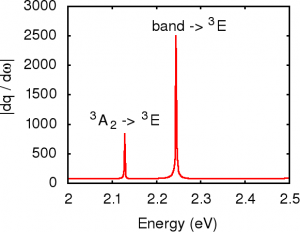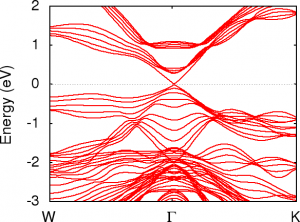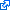The DFTB+ code is an open source project jointly developed in collaboration with the University of Bremen. This is a fast semi-empirical solid state and molecular simulation tool implementing the density functional based tight binding approximation  to DFT. Its applications are in simulating ground, excited and transport properties of molecules, nano-structures and materials.

### Some of its key features are

• Non-orthogonal tight-binding with a minimal sp (d,f…) basis
• Self-consistent and spin polarized calculations
• Standard DFT-like properties including thermodynamic and electrical levels, vibrational modes/intensities/Raman cross-sections, …
• LDA+U like corrections for strongly correlated electrons
• Spin-orbit and non-collinear spin
• Electron transport simulation through nano-structures
• Excited state calculations (time dependent DFTB)

### Key ideas

The equations for DFTB come from making an expansion around the density functional energy for a reference system. This is chosen to be a set of neutral atoms in a condensed phase environment. The non-self consistent hamiltonian, $$H^0$$, is constructed from interactions between the atomic orbitals on pairs of atoms, while the double-counting terms are grouped together into a short range pairwise repulsive potential, $$E^{rep.}(R_{AB})$$. DFTB then solves a set of Kohn-Sham like single particle equations for the quantum mechanical states of the system, and uses this to construct the single particle density matrix $$\rho$$. The atomic orbitals on neighbouring atoms are not orthogonal, so the hamiltonian and energy are given by:
\begin{aligned} H^0_{jk} c_{ik} =& \;\varepsilon_i S_{jk} c_{ik} \\ \rho_{jk} =& \sum\limits_{i} f(\varepsilon_i) c_{ij} c_{ik}\\ E =& \;Trace \left(\rho H^0\right) + \sum\limits_{ab} E^{rep.}\left(R_{ab}\right) \end{aligned}
where $$f(\varepsilon_i)$$ is the occupation of state $$i$$ which has a single particle energy $$\varepsilon_i$$.

DFTB is usually applied in its self-consistent form (SCC-DFTB or DFTB2), where the effects of charge transfer (and spin polarization when relevant) are included in equations which must now be solved iteratively. Terms in the fluctuation of charge (and magnetisation) from the neutral reference system are included.

### Some typical applications are shown belowSimulated absorption of the NV- defect in diamond

In diamond crystals, a  common defect is a complex between a missing “vacant” site and a nitrogen impurity. These VN centres have some remarkable properties.Surface states on the topological insulator Bi2Se3

Topological insulators are a new category of materials which are electrically insulating inside while being guaranteed to be conductive on the surface.

## ReferencesB. Aradi, B. Hourahine, and T. Frauenheim, “DFTB+, a sparse matrix-based implementation of the DFTB method,” Journal of Physical Chemistry A, vol. 111, iss. 26, p. 5678–5684, 2007.
[Bibtex]
@Article{strathprints31166,
author = {B. Aradi and B. Hourahine and Th. Frauenheim},
title = {DFTB+, a sparse matrix-based implementation of the DFTB method},
journal = {Journal of Physical Chemistry A},
year = {2007},
volume = {111},
number = {26},
pages = {5678--5684},
month = {July},
abstract = {A new Fortran 95 implementation of the DFTB (density functional-based tight binding) method has been developed, where the sparsity of the DFTB system of equations has been exploited. Conventional dense algebra is used only to evaluate the eigenproblems of the system and long-range Coulombic terms, but drop-in O(N) or O(N-2) modules are planned to replace the small code sections that these entail. The developed sparse storage structure is discussed in detail, and a short overview of other features of the new code is given.},
doi = {10.1021/jp070186p},
keywords = {electronic structur calculations, density functional theory, tight binding method, ewald sums, convergence, simulations, potentials, molecules, Physics, Physical and Theoretical Chemistry},
url = {http://strathprints.strath.ac.uk/31166/}
}